﻿ Inset Method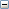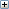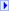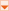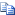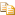Collapse All Expand All
dx
Required. A Double that represents the distance in the X direction to decrease the width of the rectangle.
dy
Required. A Double that represents the distance in the Y direction to decrease the width of the rectangle.
 Rectangle Object : Inset Method

Glossary Item Box

#Description

Decreases the width and height of the rectangle by the specified amount, in units of the coordinate system of the current map.

#Syntax

`object.Inset ( dX, dY )`

#Parameters

dx
Required. A Double that represents the distance in the X direction to decrease the width of the rectangle.
dy
Required. A Double that represents the distance in the Y direction to decrease the width of the rectangle.

#Remarks

The Inset method decreases the rectangle by deltaX at both the left and the right and decreases the rectangle by deltaY at both the top and the bottom.

#Example

Zooms in on the map by insetting the map extent
Inset Example (VBScript)Copy Code
```Sub ZoomInByInset
Dim objRect, dblDeltaX, dblDeltaY
'Set the rectangle to the current map extent
Set objRect = Application.Map.Extent
'Calculate the inset relative the current map extent
dblDeltaX = CDbl(objRect.Width / 3)
dblDeltaY = CDbl(objRect.Height / 3)
'Inset the rectangle
Call objRect.Inset (dblDeltaX, dblDeltaY)
'Re-set the map extent to the rectangle
Application.Map.Extent = objRect
Set objRect = Nothing
End Sub```